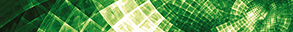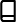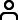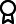Journal metrics
Acceptance rate22%
Submission to final decision43 days
Acceptance to publication32 days
CiteScore0.500
Journal Citation Indicator0.690
Impact Factor0.971

### A Two-Stage Estimator for Change Point in the Mean of Panel Data

####Journal profile

Journal of Mathematics is a broad scope journal that publishes original research and review articles on all aspects of both pure and applied mathematics.

####Editor spotlight

Chief Editor, Professor Jen-Chih Yao, is currently based at Zhejiang Normal University in China. His current research includes dynamic programming, mathematical programming, and operations research.

####Special Issues

We currently have a number of Special Issues open for submission. Special Issues highlight emerging areas of research within a field, or provide a venue for a deeper investigation into an existing research area.

## Latest Articles

More articles
Research Article

### -Anonymity in Wheel-Related Social Graphs Measured on the Base of -Metric Antidimension

For the study and valuation of social graphs, which affect an extensive range of applications such as community decision-making support and recommender systems, it is highly recommended to sustain the resistance of a social graph to active attacks. In this regard, a novel privacy measure, called the -anonymity, is used since the last few years on the base of -metric antidimension of in which is the maximum number of attacker nodes defining the -metric antidimension of for the smallest positive integer . The -metric antidimension of is the smallest number of attacker nodes less than or equal to such that other nodes in cannot be uniquely identified by the attacker nodes. In this paper, we consider four families of wheel-related social graphs, namely, Jahangir graphs, helm graphs, flower graphs, and sunflower graphs. By determining their -metric antidimension, we prove that each social graph of these families is the maximum degree metric antidimensional, where the degree of a vertex is the number of vertices linked with that vertex.

Research Article

### Observer-Based Fuzzy Synchronization and Output Tracking Control of Time-Varying Delayed Chaotic Systems

Tracking control for the output using an observer-based fuzzy synchronization of time-varying delayed discrete- and continuous-time chaotic systems is proposed in this paper. First, from a practical point of view, the chaotic systems here consider the influence of time-varying delays, disturbances, and immeasurable states. Then, to facilitate a uniform control design approach for both discrete- and continuous-time chaotic systems, the dynamic models along with time-varying delays and disturbances are reformulated using the T-S (Takagi–Sugeno) fuzzy representation. For control design considering immeasurable states, a fuzzy observer achieves master-slave synchronization. Third, combining both a fuzzy observer for state estimation and a controller (solved from generalized kinematic constraints) output tracking can be achieved. To make the design more practical, we also consider differences of antecedent variables between the plant, observer, and controller. Finally, using Lyapunov’s stability approach, the results are sufficient conditions represented as LMIs (linear matrix inequalities). The contributions of the method proposed are threefold: (i) systemic and unified problem formulation of master-slave synchronization and tracking control for both discrete and continuous chaotic systems; (ii) practical consideration of time-varying delay, immeasurable state, different antecedent variables (of plant, observer, and controller), and disturbance in the control problem; and (iii) sufficient conditions from Lyapunov’s stability analysis represented as LMIs which are numerically solvable observer and controller gains from LMIs. We carry out numerical simulations on a chaotic three-dimensional discrete-time system and continuous-time Chua’s circuit. Satisfactory numerical results further show the validity of the theoretical derivations.

Research Article

### On the Recurrent -Semigroups, Their Existence, and Some Criteria

In this paper, recurrent -semigroups are introduced and investigated. It is proved that, despite hypercyclic -semigroups, recurrent -semigroups can be found on finite-dimensional Banach spaces. Some criteria are stated for recurrence, which is based on open sets, neighborhoods of zero, and special eigenvectors. It is established that having a dense set of recurrent vectors is a sufficient and necessary condition for a -semigroup to be recurrent. Moreover, the direct sum of recurrent -semigroups is investigated.

Research Article

### Complex Neutrosophic Soft Matrices Framework: An Application in Signal Processing

In this paper, we introduce the concept of complex neutrosophic soft matrices. We define some basic operations including complement, union, and intersection on these matrices. We extend the concept of complex neutrosophic soft sets to complex neutrosophic soft matrices and prove related properties. Moreover, we develop an algorithm using complex neutrosophic soft matrices and apply it in signal processing.

Research Article

### Dynamic Analysis and Hopf Bifurcation of a Lengyel–Epstein System with Two Delays

In this paper, a Lengyel–Epstein model with two delays is proposed and considered. By choosing the different delay as a parameter, the stability and Hopf bifurcation of the system under different situations are investigated in detail by using the linear stability method. Furthermore, the sufficient conditions for the stability of the equilibrium and the Hopf conditions are obtained. In addition, the explicit formula determining the direction of Hopf bifurcation and the stability of bifurcating periodic solutions are obtained with the normal form theory and the center manifold theorem to delay differential equations. Some numerical examples and simulation results are also conducted at the end of this paper to validate the developed theories.

Research Article

### Multiple Solutions for Second-Order Sturm–Liouville Boundary Value Problems with Subquadratic Potentials at Zero

We deal with the following Sturm–Liouville boundary value problem: Under the subquadratic condition at zero, we obtain the existence of two nontrivial solutions and infinitely many solutions by means of the linking theorem of Schechter and the symmetric mountain pass theorem of Kajikiya. Applying the results to Sturm–Liouville equations satisfying the mixed boundary value conditions or the Neumann boundary value conditions, we obtain some new theorems and give some examples to illustrate the validity of our results.Journal metrics
Acceptance rate22%
Submission to final decision43 days
Acceptance to publication32 days
CiteScore0.500
Journal Citation Indicator0.690
Impact Factor0.971Author guidelinesEditorial boardDatabases and indexingAuthor guidelinesEditorial boardDatabases and indexing

Article of the Year Award: Outstanding research contributions of 2020, as selected by our Chief Editors. Read the winning articles.